# NCERT Solutions for Class 10 Maths Chapter 11 Constructions Ex 11.1

Get Free NCERT Solutions for Class 10 Maths Chapter 11 Ex 11.1 PDF. Constructions Class 10 Maths NCERT Solutions are extremely helpful while doing your homework. Exercise 11.1 Class 10 Maths NCERT Solutions were prepared by Experienced ncert-books.in Teachers. Detailed answers of all the questions in Chapter 11 Maths Class 10 Constructions Exercise 11.1 provided in NCERT TextBook.Question 1.
Draw a line segment of length 7.6 cm and divide it in the ratio 5:8. Measure the two parts.
Solution:Question 2.
Construct a triangle of sides 4 cm, 5 cm and 6 cm and then a triangle similar to it whose sides are$\frac { 2 }{ 3 }$of the corresponding sides of the first triangle.
Solution: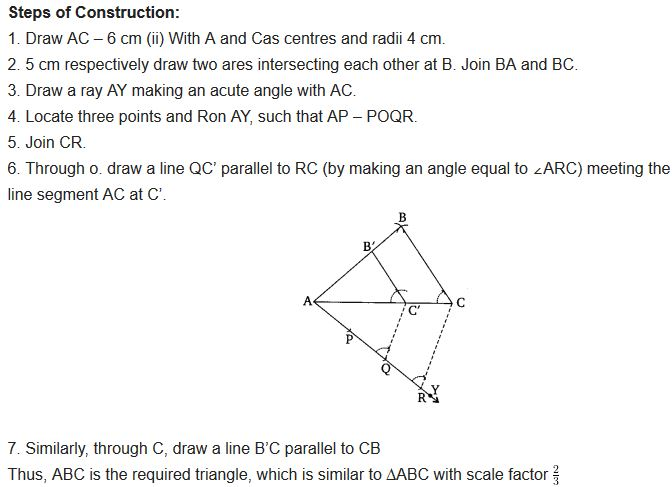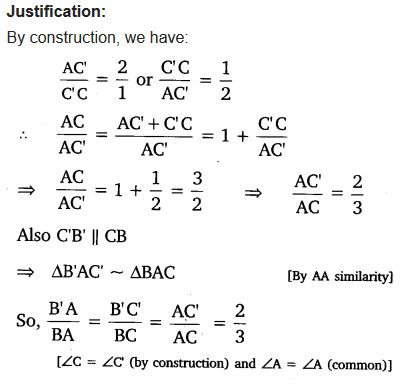Question 3.
Construct a triangle with sides 5 cm, 6 cm, and 7 cm and then another triangle whose sides are$\frac { 7 }{ 5 }$of the corresponding sides of the first triangle.
Solution: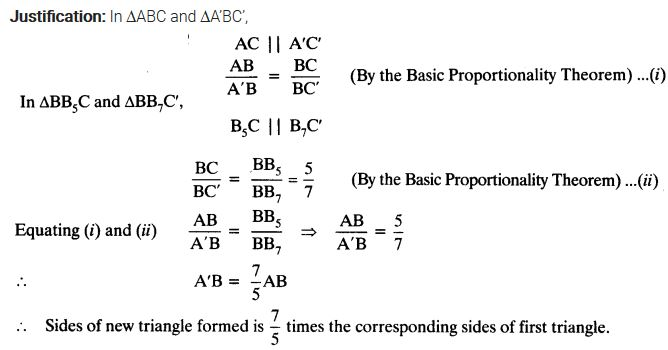Question 4.
Construct an isosceles triangle whose base is 8 cm and altitude 4 cm and then another triangle whose sides are 1$\frac { 1 }{ 2 }$times the corresponding sides of the isosceles triangle.
Solution:Question 5.
Draw a triangle ABC with side BC = 6 cm, AB = 5 cm and ∠ABC = 60°. Then construct a triangle whose sides are$\frac { 3 }{ 4 }$of the corresponding sides of the triangle ABC.
Solution:Question 6.
Draw a triangle ABC with side BC = 7 cm, ∠B = 45°, ∠A = 105°. Then, construct a triangle whose sides are$\frac { 4 }{ 3 }$times the corresponding sides of ∆ABC.
Solution:Question 7.
Draw a right triangle in which the sides (other than hypotenuse) are of lengths 4 cm and 3 cm. Then construct another triangle whose sides are$\frac { 5 }{ 3F }$times the corresponding sides of the given triangle.
Solution: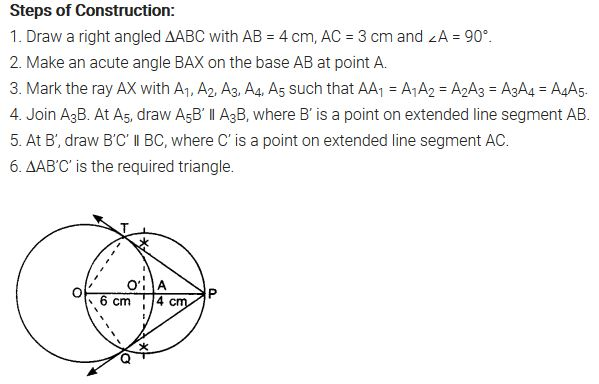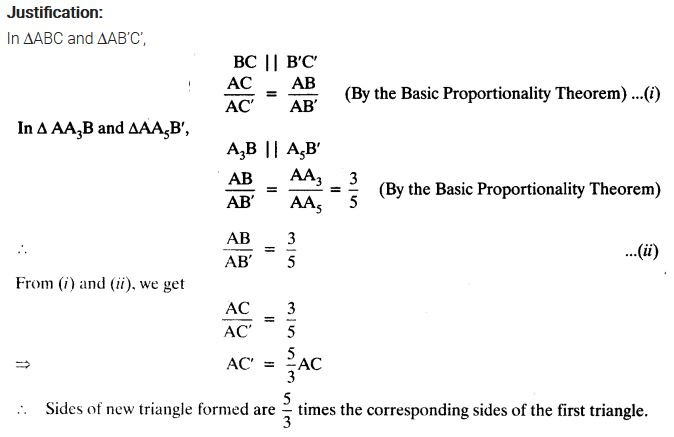error: Content is protected !!
+